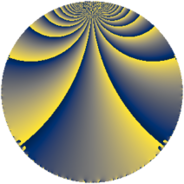# Properties

 Label 96.3.mLevel $96$ Weight $3$ Character orbit 96.m Rep. character $\chi_{96}(19,\cdot)$ Character field $\Q(\zeta_{8})$ Dimension $64$ Newform subspaces $1$ Sturm bound $48$ Trace bound $0$

# Related objects

## Defining parameters

 Level: $$N$$ $$=$$ $$96 = 2^{5} \cdot 3$$ Weight: $$k$$ $$=$$ $$3$$ Character orbit: $$[\chi]$$ $$=$$ 96.m (of order $$8$$ and degree $$4$$) Character conductor: $$\operatorname{cond}(\chi)$$ $$=$$ $$32$$ Character field: $$\Q(\zeta_{8})$$ Newform subspaces: $$1$$ Sturm bound: $$48$$ Trace bound: $$0$$

## Dimensions

The following table gives the dimensions of various subspaces of $$M_{3}(96, [\chi])$$.

Total New Old
Modular forms 136 64 72
Cusp forms 120 64 56
Eisenstein series 16 0 16

## Trace form

 $$64q + O(q^{10})$$ $$64q + 40q^{10} + 48q^{12} + 32q^{14} - 8q^{16} - 24q^{18} - 160q^{20} - 184q^{22} + 128q^{23} - 72q^{24} - 200q^{26} - 120q^{28} + 40q^{32} + 120q^{34} - 192q^{35} + 280q^{38} + 584q^{40} - 192q^{43} + 104q^{44} + 32q^{46} - 312q^{50} - 192q^{51} - 424q^{52} + 320q^{53} - 72q^{54} - 256q^{55} - 392q^{56} - 352q^{58} - 256q^{59} - 144q^{60} + 64q^{61} - 48q^{62} + 408q^{64} + 144q^{66} + 64q^{67} + 856q^{68} - 192q^{69} + 984q^{70} + 512q^{71} + 1056q^{74} + 384q^{75} + 296q^{76} - 448q^{77} + 360q^{78} + 512q^{79} + 328q^{80} - 760q^{82} - 448q^{86} - 1072q^{88} + 192q^{91} - 784q^{92} - 480q^{94} + 600q^{96} + 272q^{98} + O(q^{100})$$

## Decomposition of $$S_{3}^{\mathrm{new}}(96, [\chi])$$ into newform subspaces

Label Dim. $$A$$ Field CM Traces $q$-expansion
$$a_2$$ $$a_3$$ $$a_5$$ $$a_7$$
96.3.m.a $$64$$ $$2.616$$ None $$0$$ $$0$$ $$0$$ $$0$$

## Decomposition of $$S_{3}^{\mathrm{old}}(96, [\chi])$$ into lower level spaces

$$S_{3}^{\mathrm{old}}(96, [\chi]) \cong$$ $$S_{3}^{\mathrm{new}}(32, [\chi])$$$$^{\oplus 2}$$